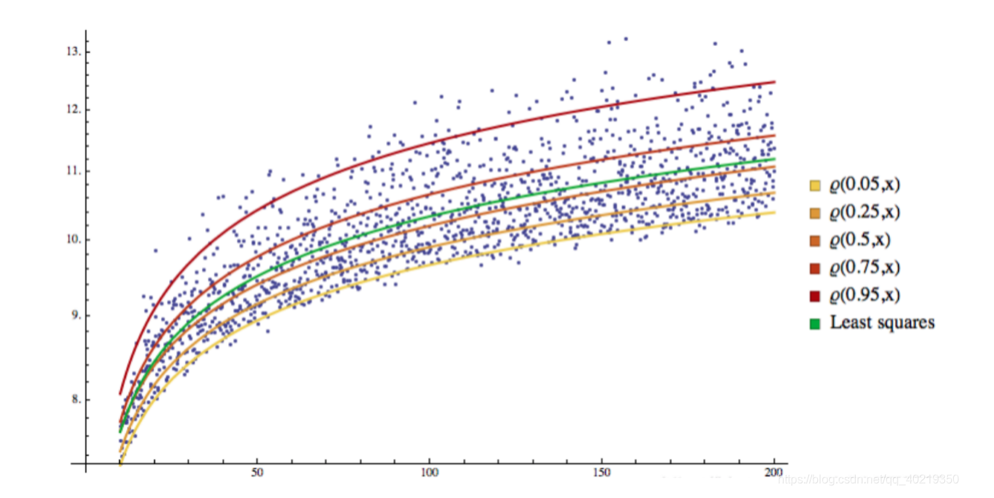# GARCH-QR非线性回归(GQNR)交易模型

Author: Benson, Created: 2021-04-21 00:30:43, Updated: 2021-04-26 10:42:18

# 一、前言

## 量化交易的优势之处

量化交易是指以先进的数学模型替代人为的主观判断，利用计算机技术从庞大的历史数据中海选能带来超额收益的多种“大概率”事件以制定策略，极大地减少了投资者情绪波动的影响，避免在市场极度狂热或悲观的情况下作出非理性的投资决策。         由于数字货币24*7小时不间断的交易市场的连续性，并且量化交易可以达到高频交易的效果，从数字货币市场入手显然是做量化的很好的起步点。目前数字货币市场仍然是不成熟的。平台交易系统的宕机，k线插针依然是会偶尔出现，对于量化交易也是一种风险所在。不过对于数字货币进行量化交易总体来看依然是利大于弊。因为通过模型的回测训练和时间序列的回测分析，我们可以在最短时间能尝试到数百种模型中最合适的方式。

# 二、GQNR模型简介

本模型是基于Garch模型预测波动率，通过分位数回归预测波动率的VaR值进而利用非线性回归，例如GA进行拟合来预测未来下一个周期中的上限VaR以及下限VaR，在后文中将这种方法模型简称为GQNR。

## 1.Garch模块

本板块将会详细介绍策略Garch核心的推导，此方法在金融市场具有一定的普适性，在数字货币上能到达一定预测效果。

### 1.1Garch定义

ARCH模型的实质是使用残差平方序列的q阶移动平移拟合当期异方差函数值，由于移动平均模型具有自相关系数q阶截尾性，所以ARCH模型实际上只适用于异方差函数短期自相关系数。         但是在实践中，有些残差序列的异方差函数是具有长期自关性，这时使用ARCH模型拟合异方差函数，将会产生很高的移动平均阶数，增加参数估计的难度并最终影响ARCH模型的拟合精度。         为了修正个问题，提出了广义自回归条件异方差模型， 这个模型简记为GARCH（p,q）.         GARCH模型实际上就是在ARCH的基础上，增加考虑异方差函数的p阶自回归性而形成，它可以有效的拟合具有长期记忆性的异方差函数。ARCH模型是GARCH模型的一个特例，p=0的GARCH（p,q）模型。

### 1.2ARCH过程

定义σn是在第n-1个交易周期估计资产在第n个交易周期的波动率，mu为日收益率，那么可以根据最近m个交易周期的收益率进行无偏估计： $$\sigma n^2= \frac{1}{m-1} \sum\limits{i=1}^m {( { \mu_{n-i}- \overline{\mu} } ) ^2},$$         做以下变化①将μn-i换成百分比收益率；②将m-1换成m；③假设μ=0,并且这些变化对结果影响不大，根据上式可以将波动率简化为： $$\sigma n^2= \frac{1}{m} \sum\limits{i=1}^m { \mu_{n-i} ^2},$$         也就是说每一个周期的波动率的平方具有等权重1/m，由于是估计当前的波动率，距离近的数据应该赋予更高权重，则上式可以更改为： $$\sigma n^2= \sum\limits{i=1}^m { \alpha_i\mu_{n-i} ^2},$$         αi是第i个交易周期的收益率平方的系数，取正值且i越小值越大，权重之和为1。进一步推广，假定存在一个长期的方差率VL，且对应的权重为γ，根据上式可以得到：

$$\begin{cases}\sigma n^2= \gamma V{L}+\sum\limits_{i=1}^m { \alpha_i\mu_{n-i} ^2}\ &\ \gamma+\sum\limits_{i=1}^m{\alpha_i\mu_{n-i}^2}=1 & \end{cases} ,$$         令ω=γVL，公式(15)可以改写为： $$\sigma n^2= \omega+\sum\limits{i=1}^m { \alpha_i\mu_{n-i} ^2},$$         根据上式我们可以得到常见的ARCH(1)过程 $$\sigma n^2= \omega+{ \alpha\mu{n-1} ^2},$$

### 1.3GARCH过程

GARCH(p,q)模型是ARCH§和EWMA(q)模型的结合，意义为波动率不仅与前p期收益有关，还与自身前q期有关，表达式如下： $$\sigma n^2= \omega+\sum\limits{i=1}^m { \alpha_i\mu_{n-i} ^2}+\sum\limits_{i=1}^m { \beta_i\sigma_{n-i} ^2},$$         根据上式我们可以得到常见的GARCH(1,1)： $$\begin{cases}\sigma n^2= \omega+{ \alpha\mu{n-1} ^2+\beta\sigma_{n-1}^2}\&\ \qquad\alpha+\beta+\gamma=1 & \end{cases} ,$$

## 2 QR模块

本板块将会阐述基本的分位数回归，描述策略性分位数的重要性

### 2.2 由OLS到QR

一般的回归方法是最小二乘法，即最小化误差的平方和： $$min \sum{({y_i- \widehat{y}i })}^2$$        而分位数的目标就是在上述公式基础上最小化加权的误差绝对值和： $$\mathop{\arg\min\beta}\ \ \sum{[{\tau(y_i-X_i\beta)^++(1-\tau)(X_i\beta-y_i) ^+ }]}$$

### 2.2 QR可视化

可以看到，所有样本被回归线分成了不同的空间，而这个回归线也就成了分位线## 3.GARCH-QR回归

我们很自然联想到，是否可以用市场未知的波动率sigma与分位数Q即VaR进行回归来预测未来大概率情况下波动阈值，本板块将进行这一方向的展开。

### 3.1 选定波动率与VaR的回归形式

由于此处涉及策略核心，我暂且举一个形式，以说明思路。 $$VaR=\epsilon+W^TE\E=(\zeta,\zeta^2,\zeta^3,\zeta^4)\W=(W_1,W_2,W_3,W_4)$$

### 3.2 确定目标函数

根据以上信息，我们进行组合后可以得到最终待优化的目标函数： $$\widehat{W}=\mathop{\arg\min_W}\ \ \sum{[{\alpha(VaR_t-W^TE_t)^++(1-\alpha)(W^TE_t-VaR_t) ^+ }]}$$

### 3.3 使用机器学习进行目标函数的优化

本步骤可选性比较多，传统的梯度下降，也可以遗传算法，读者可以发挥自己的创意进行实验。本处采取的是优化后的GA算法，曾在另一篇自己的blog里面详细介绍过不再展开，有关于GA算法地址

# 三、如何在量化中运用GQNR

## 1.思路的确定

GQNR的核心在于市场的波动率，在每一期当前的时间点上，可以通过GARCH预测出对于下一期波动率的预测，在另一方面，通过过往数据预测波动率的分位数回归，能够获得在大概率情况下不会超出的波动阈值上边界与下边界。而这两个边界，就是整体的核心。一旦触发上边界，我们可以认为在大概率下短期会有回调的趋势，一旦触发下边界，我们可以认为在大概率情况下短期会有拉升趋势。

• 采取回归的形式
• 适应性算法的选择
• 机器学习合适的参数
• 市场的不确定随机性

## 3.解决方案

• 减短策略学习的时间周期
• 降低单笔下仓保证金抵抗长期风险
• 增加双均线趋势共同验证以及二次阈值确认

More

Benson 当前的波动率就一个数据点，拿什么回归，如果拿历史数据点一起回归，那也只能做OLS，不能做分位数回归